### 温标之间的关系

2021-08-23       来源：www.weilianchina.com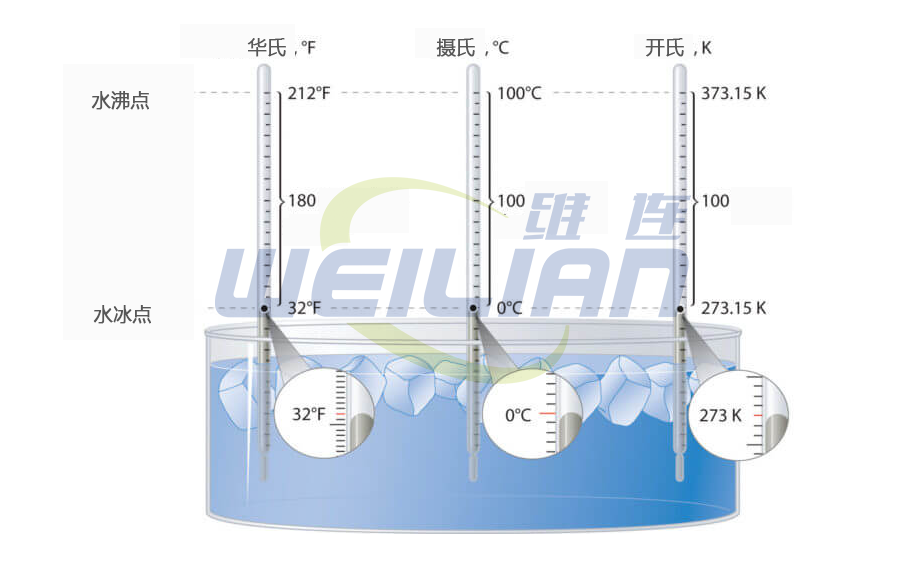温标之间的关系 温标之间的关系

##### 为了确定华氏度和摄氏度之间的关系，我们可以绘制 F（温度单位为°F）与 C（温度单位为°C）的图表，其中 C 的范围为 0 ≤ C ≤ 100。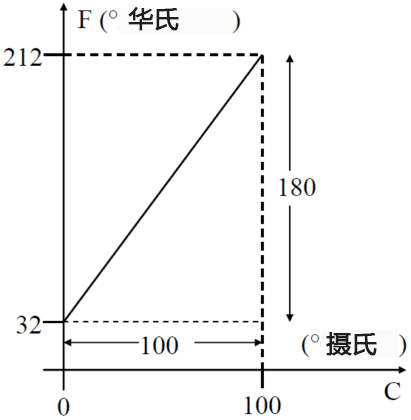y = mx + c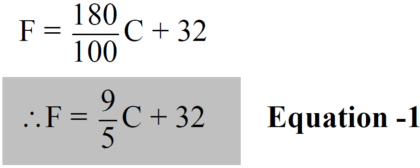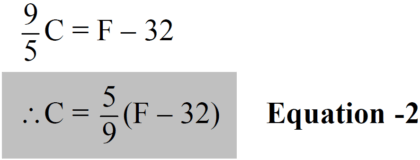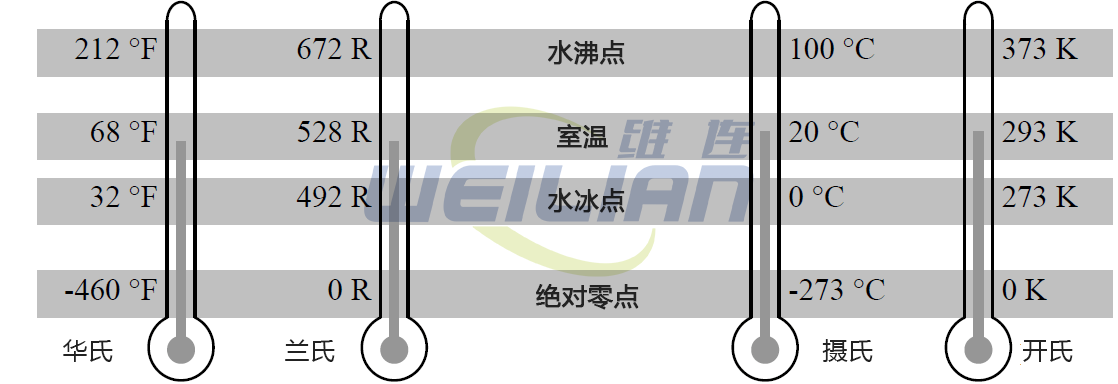F = (9/5)C + 32 = (9/5)×(-273.15) + 32 = -459.67 °F ≈ -460 °F

C = (5/9)(F – 32) = (5/9)×(86 – 32) = 30 °C

R = F + 460 = 86 + 460 = 546 R

K = C + 273 = 30 + 273 = 303 K

F = (5/9)(F – 32) ⇒ 9F = 5F – 160 ⇒ 4F = -160 ⇒ F = -40 °F

C = (5/9)(F – 32) = (5/9)(0 – 32) = -17.78 °C维连在线客服
>
Top